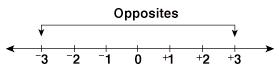# Integer Properties and Arithmetic

Integer properties and arithmetic are presented in this unit. Try our integer lessons below, or browse other units of instruction.Integer Unit Description Introduction To understand integer properties including opposites, positive, negative, number line, and signs. To read and write signed numbers. To connect integer concepts with real-life situations. Absolute Value To understand the concept of absolute value and how it relates to the number line. To use proper notation. Compare and Order To order a set of signed numbers from least to greatest, and from greatest to least -- with and without the number line. Integer Addition Absolute value is a pre-requisite for this lesson. To learn integer addition with like and unlike signs. Integer Subtraction To subtract an integer by adding its opposite. Problems with like and unlike signs are presented. Integer Multiplication To learn integer multiplication with like signs and unlike signs. Students will learn to multiply up to three factors. Integer Division To learn integer division with like and unlike signs. Operations with Integers To review and apply the rules for all four operations (mixed review format). To apply integer arithmetic concepts. Practice Exercises To complete 10 additional exercises as practice. To assess students' understanding of all integer properties and arithmetic presented. Challenge Exercises To solve 10 additional problems that challenge students' understanding of integer properties and arithmetic. All problems make connections to the real world. To hone students' problem-solving skills. Solutions To review complete solutions to all exercises presented in this unit. Includes the problem, step-by-step solutions, and final answers for each exercise. Learning Objectives Introduction, absolute value, comparing and ordering, adding, subtracting, multiplying, dividing, and all four operations.